# The VARMAX Procedure

### OUTSTAT= Data Set

The OUTSTAT= data set contains estimation results of the fitted model produced by the VARMAX statement. The following output variables can be created. The subindex i is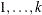, where k is the number of endogenous variables.

• the BY variables

• NAME, a character variable that contains the name of endogenous (dependent) variables

• SIGMA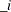, numeric variables that contain the estimate of the innovation covariance matrix

• AICC, a numeric variable that contains the corrected Akaike’s information criterion value

• HQC, a numeric variable that contains the Hannan-Quinn’s information criterion value

• AIC, a numeric variable that contains the Akaike’s information criterion value

• SBC, a numeric variable that contains the Schwarz Bayesian’s information criterion value

• FPEC, a numeric variable that contains the final prediction error criterion value

• FValue, a numeric variable that contains the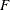statistics

• PValue, a numeric variable that contains p-value for thestatistics

If the JOHANSEN= option is specified, the following items are added:

• Eigenvalue, a numeric variable that contains eigenvalues for the cointegration rank test of integrated order 1

• RestrictedEigenvalue, a numeric variable that contains eigenvalues for the cointegration rank test of integrated order 1 when the NOINT option is not specified

• Beta, numeric variables that contain long-run effect parameter estimates,• Alpha, numeric variables that contain adjustment parameter estimates,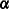If the JOHANSEN=(IORDER=2) option is specified, the following items are added:

• EValueI2, numeric variables that contain eigenvalues for the cointegration rank test of integrated order 2

• EValueI1, a numeric variable that contains eigenvalues for the cointegration rank test of integrated order 1

• Eta, numeric variables that contain the parameter estimates in integrated order 2,
• Xi, numeric variables that contain the parameter estimates in integrated order 2,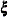The OUTSTAT= data set contains the values shown Table 35.11 for a bivariate case.

Table 35.11: OUTSTAT= Data Set

 Obs NAME SIGMA_1 SIGMA_2 AICC RSquare FValue PValue 1 y1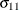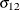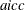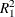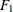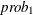2 y2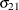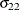.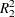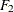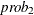Obs EValueI2_1 EValueI2_2 EValueI1 Beta_1 Beta_2 1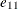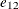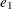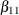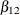2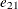.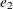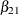Obs Alpha_1 Alpha_2 Eta_1 Eta_2 Xi_1 Xi_2 1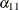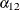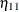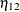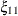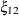2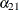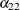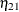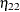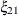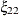Consider the following example:

proc varmax data=simul2 outstat=stat;
model y1 y2 / p=2 noint
cointtest=(johansen=(iorder=2))
ecm=(rank=1 normalize=y1)
noprint;
run;

proc print data=stat;
run;


The output in Figure 35.72 shows the results of the OUTSTAT= data set.

Figure 35.72: OUTSTAT= Data Set

Obs NAME SIGMA_1 SIGMA_2 AICC HQC AIC SBC FPEC RSquare FValue PValue EValueI2_1 EValueI2_2 EValueI1 Beta_1 Beta_2 Alpha_1 Alpha_2 Eta_1 Eta_2 Xi_1 Xi_2
1 y1 94.7557 4.527 9.37221 9.43236 9.36834 9.52661 11712.14 0.93900 482.308 6.1637E-57 0.98486 0.95079 0.50864 1.00000 1.00000 -0.46680 0.007937 -0.012307 0.027030 54.1606 -52.3144
2 y2 4.5268 109.570 . . . . . 0.93912 483.334 5.6124E-57 0.81451 . 0.01108 -1.95575 -1.33622 0.10667 0.033530 0.015555 0.023086 -79.4240 -18.3308